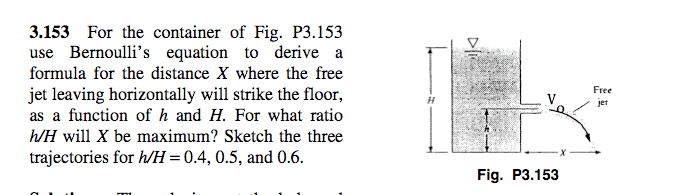# Bernoulli Equation: Finding t_fall Expression

## Homework Statement## Homework Equations

X=Vo*tfall

Bernoulli Eq: $$\frac {p}{\rho}+\frac{V^2}{2}+gz=const$$

I used B.E. to find an expression for the velocity of the exit jet:

$$V_o=\sqrt{2g(H-h)}$$

For some reason I am confused as to how to find an expression for t_fall ?

I know it should be easy, but I am under the impression I am supposed to use the B.E. to find it...

Any hints?

I know it should be easy, but I am under the impression I am supposed to use the B.E. to find it...
That's not how I read the problem. You've used B.E. to find V0. The rest is just projectile motion.

Doc Al said:
That's not how I read the problem. You've used B.E. to find V0. The rest is just projectile motion.

Oh. Easy Enough thenThanks again Doc!

t=sqrt(2h/g)